# combination logic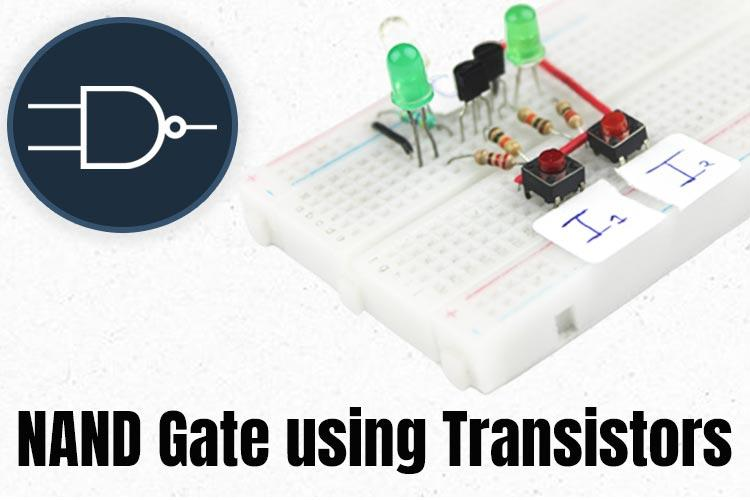How to Build a NAND Gate with Transistors?In the realm of digital electronics and logic circuits, the NAND gates stands as a…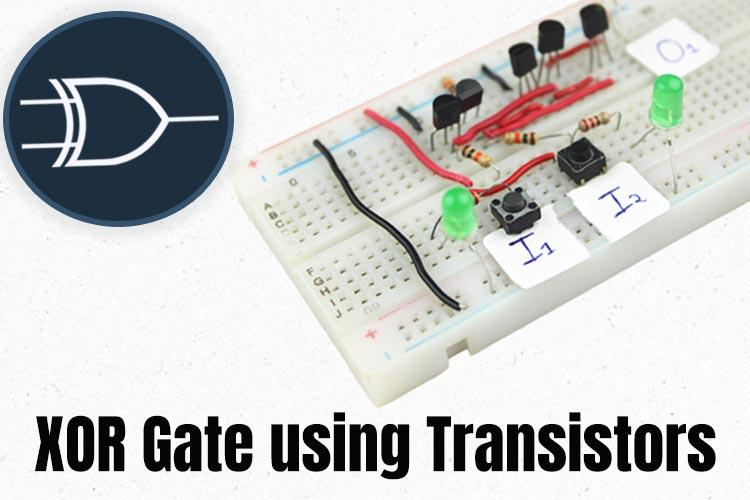How to Build an XOR Gate with Transistors?In the vast world of digital electronics and logic circuits, The XOR gate stands as a…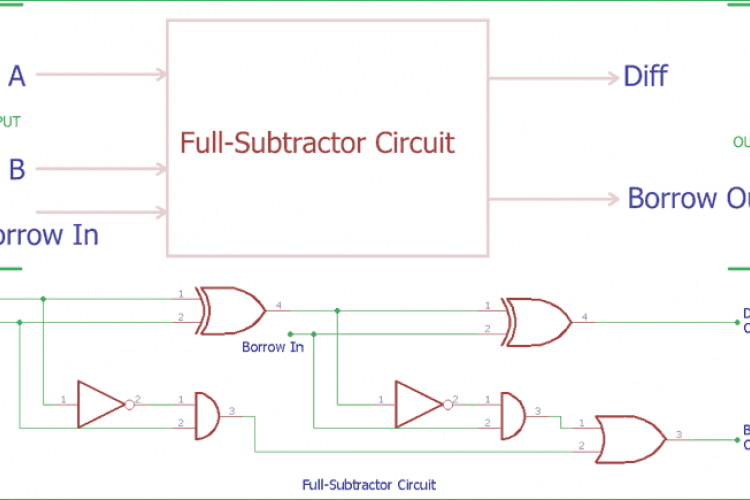Full Subtractor Circuit and Its ConstructionIn the previous tutorial of Half Subtractor Circuit, we had seen how computer use single bit binary numbers 0 and 1 for…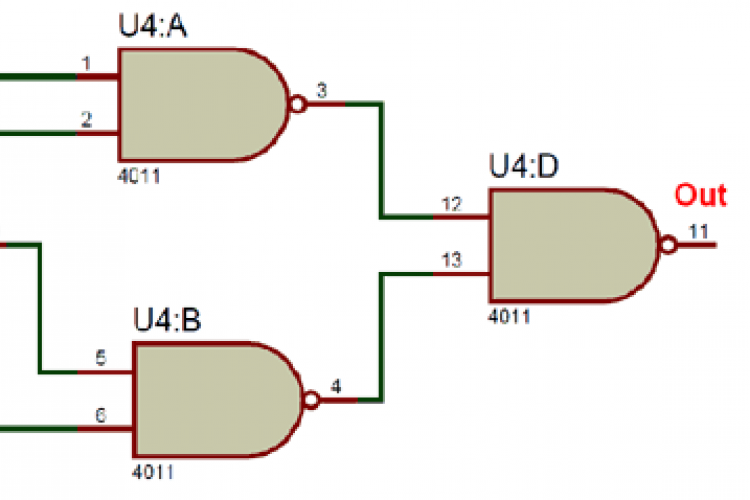Multiplexer Circuit and How it WorksThe term Multiplexer which is also commonly called as “MUX” or “MPX” refers to selecting one output of the many available…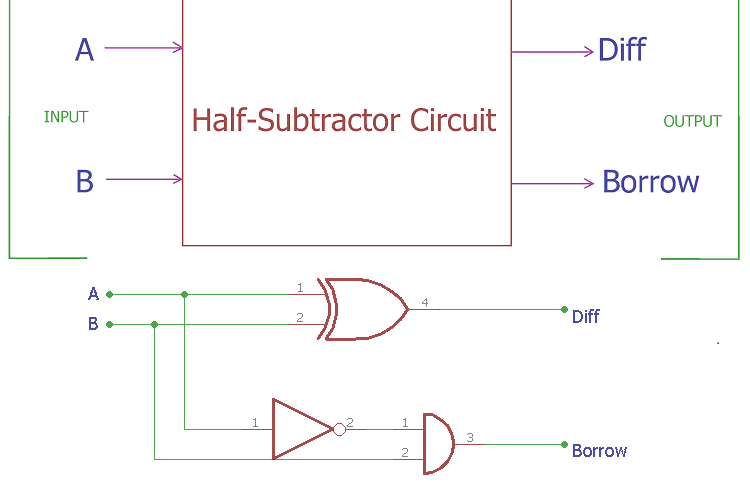Half Subtractor Circuit and Its ConstructionIn previous tutorials, we have seen how computer use binary numbers 0 and 1 and by using an adder circuit computer will add…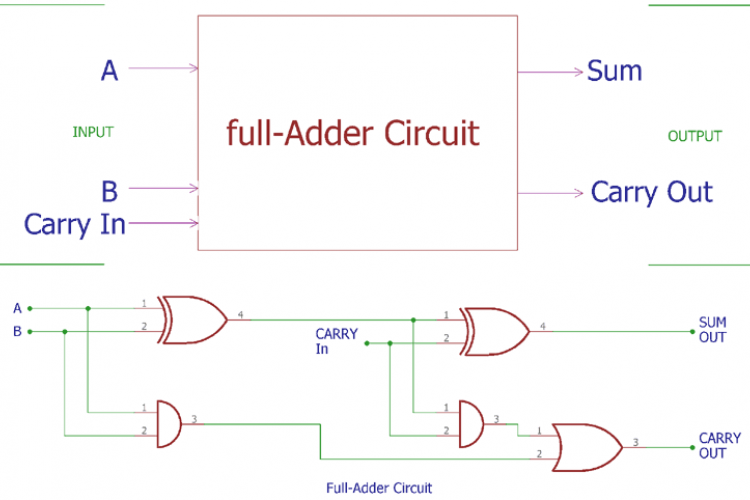Full Adder Circuit and its ConstructionIn previous tutorial of half adder circuit construction, we had seen how computer uses single bit binary numbers 0 and 1 for…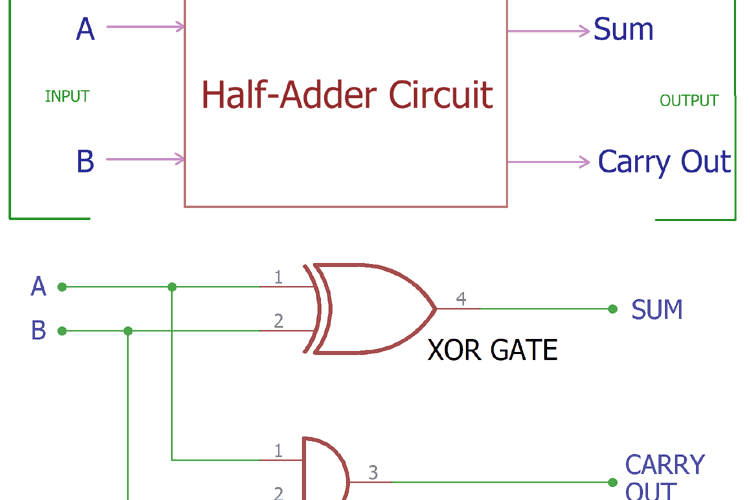Half Adder Circuit and its ConstructionComputer uses binary numbers 0 and 1. An adder circuit uses these binary numbers and calculates the addition. A binary adder…